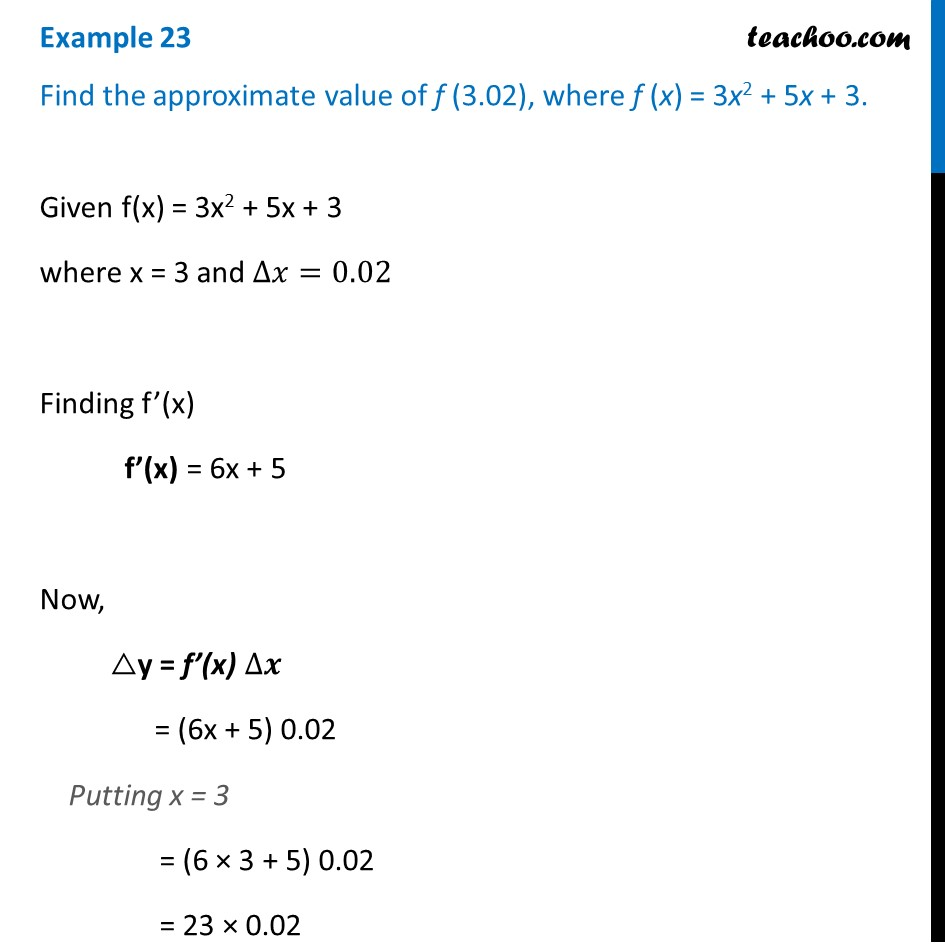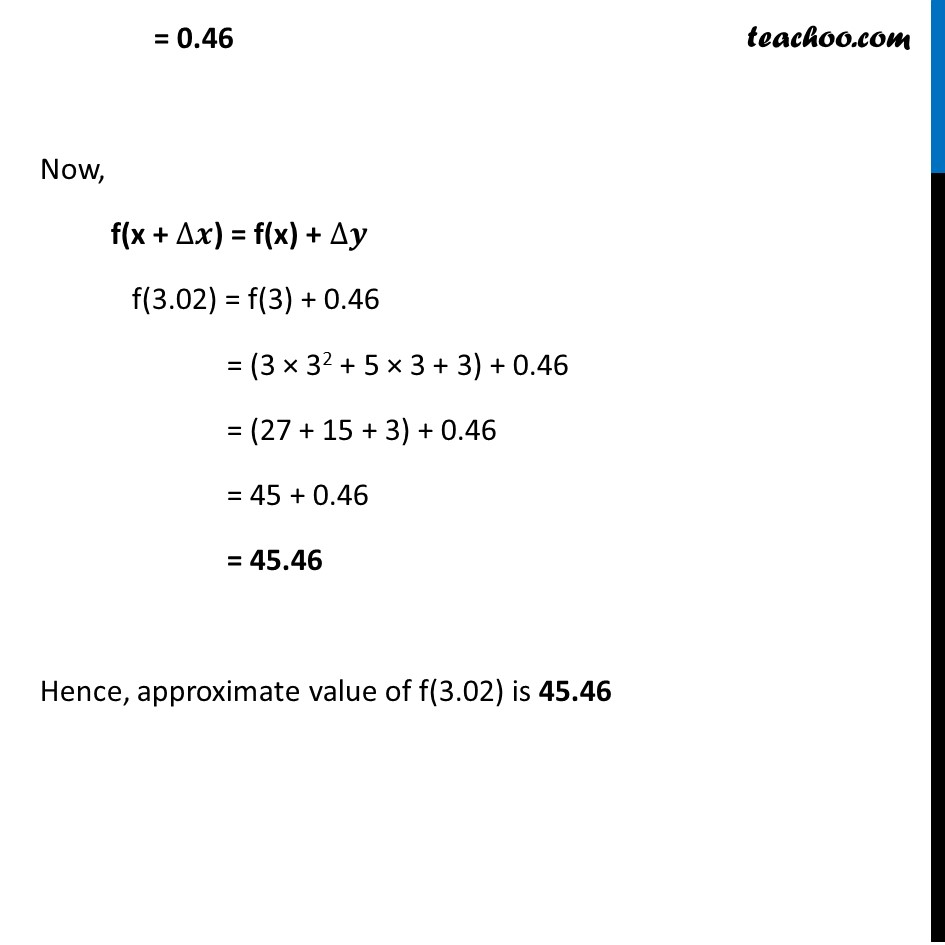Finding approximate value of function

Chapter 6 Class 12 Application of Derivatives (Term 1)
Concept wise### Transcript

Example 23 Find the approximate value of f (3.02), where f (x) = 3x2 + 5x + 3.Given f(x) = 3x2 + 5x + 3 where x = 3 and ∆𝑥=0.02 Finding f’(x) f’(x) = 6x + 5 Now, △y = f’(x) ∆𝒙 = (6x + 5) 0.02 Putting x = 3 = (6 × 3 + 5) 0.02 = 23 × 0.02 = 0.46 Now, f(x + ∆𝒙) = f(x) + ∆𝒚 f(3.02) = f(3) + 0.46 = (3 × 32 + 5 × 3 + 3) + 0.46 = (27 + 15 + 3) + 0.46 = 45 + 0.46 = 45.46 Hence, approximate value of f(3.02) is 45.46